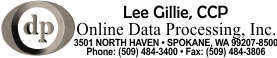# Moving some text elements on a PDF page

Q:

These are my first attempts at editing existing PDF pages. Trying to move/shift all text and images found within a rectangle by some DeltaX and DeltaY.

My code is based on ElementEdit sample that comes as part of the SDK
http://www.pdftron.com/pdfnet/samplecode.html#ElementEdit

Top half of the page it leaves text untouched / Midpoint to 3.47 inches from bottom of page is changed to blue (reminiscent of the original example)

But everything below 3.47 inches from the bottom is shifted.
The shift is 1/4" to the right (DeltaX) and down 3/4" (DeltaY).

To do this your ProcessElements Sub is recorded as:

`Private DeltaX As Double = 0.25 * 72.0`
`Private DeltaY As Double = -0.75 * 72.0`

`Sub ProcessElements(ByVal reader As ElementReader, ByVal writer As ElementWriter)`
`Dim element As Element = reader.Next()`
`While Not IsNothing(element)`
`If element.GetType() = element.Type.e_text Then`
`Dim bbox As New Rect`
`element.GetBBox(bbox)`
`If bbox.y2 < 250 Then`
`Dim mtx As Matrix2D = element.GetGState.GetTransform`
`mtx.Concat(1, 0, 0, 1, DeltaX, DeltaY)`
`element.GetGState.SetTransform(mtx)`
`writer.WritePlacedElement(element)`
`ElseIf bbox.y2 < 396 Then`
`Dim gs As GState = element.GetGState()`
`gs.SetFillColorSpace(ColorSpace.CreateDeviceRGB)`
`gs.SetFillColor(New ColorPt(0, 0, 1))`
`writer.WriteElement(element)`
`Else`
`writer.WriteElement(element)`
`End If`
`element = reader.Next()`
`ElseIf element.GetType() = element.Type.e_form Then`
`reader.FormBegin()`
`ProcessElements(reader, writer)`
`reader.End()`
`writer.WriteElement(element)`
`element = reader.Next()`
`ElseIf element.GetType() = element.Type.e_image Then`
`element = reader.Next()`
`ElseIf element.GetType() = element.Type.e_inline_image Then`
`element = reader.Next()`
`Else`
`writer.WriteElement(element)`
`element = reader.Next()`
`End If`
`End While`
`End Sub`

The input and output PDF are attached.

although (slightly) visible, you can see all the elements bunched up into the lower left corner of the page, similar placement to what I am seeing.

## Hoping taking from your sample, and providing input and output files will make it easier for you to repro, and visualize the result.

A:

For your application, WriteElement will give the wrong result, since each element will add an additional translation, resulting in a ‘staircase’ effect.

WritePlacedElement also gives the wrong result, because you end up discarding relevant GState information, such as selected font and text matrix. Instead, what you need is to only reset the GState transform, not the entire GState.

I wrote a test implementation in python that does just that. It should be relatively straightforward to map the implementation to VB:

#We will store the inverse to our translation, so we can undo it later

inverse_transform = Matrix2D(1,0,0,1,0,0)

while element != None:

#Apply the inverse transform to undo the translation

mtx = element.GetGState().GetTransform()

mtx = inverse_transform * mtx

element.GetGState().SetTransform(mtx)

#There is no longer a translation to inverse, so we set inverse_transform back to identity

inverse_transform = Matrix2D(1,0,0,1,0,0)

type = element.GetType()

if type == Element.e_text or type == Element.e_image:

#We want to translate text and images, so here we go:

mtx.Concat(1,0,0,1,0,-150)

element.GetGState().SetTransform(mtx)

writer.WriteElement(element)

#We now need to set the inverse transform:

inverse_transform = Matrix2D(1,0,0,1,0,150)

elif type == Element.e_form: # Recursively process form XObjects

writer.WriteElement(element)

else:

writer.WriteElement(element)

To help you with debugging, I would recommend you use a tool such as CosEdit, which allows you to browse the internal structure of a pdf document: http://www.pdftron.com/pdfcosedit

With this tool, and a good understanding of the PDF specification, you can easily visualize how different routines are modifying the PDF, which should make your development process more productive.

I am doing this very thing, using your approach, and as closely as possible. This works well 80% of the time for me. Most of the target text is displaced vertically by 1.0" as desired.

But there is a portion of the input document whose text just seems to disappear from the output. I am thinking because of structured elements or the use of forms there may be more I need to do than is shown in your answer? I suspect the problem lies somewhere in the input document structure (which I have no control over). And that extra steps may be needed in my ProcessElements routine.

Here is the structure I see for the portion that vanishes…

`*Nothing after this point appears in the output document...*`

`Element(e_group_begin:)`
`Element(e_form:Rect(x1=0,x2=8.5,y1=0,y2=11))`
`Element(e_group_begin:)`
`Element(e_form:Rect(x1=1.57606944444444,x2=2.42531944444444,y1=8.65619402777778,y2=8.86541625))`
`Element(e_marked_content_begin:)`
`Element(e_group_begin:)`
`Element(e_group_begin:)`
`Element(e_path:Rect(x1=1.57606944444444,x2=2.42531944444444,y1=8.65619402777778,y2=8.86541625))`
`Element(e_path:Rect(x1=1.57606944444444,x2=2.42531944444444,y1=8.65619402777778,y2=8.86541625))`
`Element(e_group_begin:)`
`Element(e_path:Rect(x1=1.58995833333333,x2=2.41143055555556,y1=8.67008291666667,y2=8.85152736111111))`
`Element(e_group_begin:)`
`Element(e_text_begin:)`
`Element(e_text:Text("612"),Rect(x1=1.60384722222222,x2=1.81234722222222,y1=8.69080513888889,y2=8.80643013888889))`
`Element(e_text_end:)`
`Element(e_group_end:)`
`Element(e_group_end:)`
`Element(e_group_end:)`
`Element(e_group_end:)`
`Element(e_marked_content_end:)`
`Element(e_form:Rect(x1=3.34340291666667,x2=4.54458347222222,y1=8.65619402777778,y2=8.86541625))`
`Element(e_group_begin:)`
`Element(e_marked_content_begin:)`
`Element(e_group_begin:)`
`Element(e_path:Rect(x1=3.34340291666667,x2=4.54458347222222,y1=8.65619402777778,y2=8.86541625))`
`Element(e_path:Rect(x1=3.34340291666667,x2=4.54458347222222,y1=8.65619402777778,y2=8.86541625))`
`Element(e_group_begin:)`
`Element(e_path:Rect(x1=3.35729180555556,x2=4.53069458333333,y1=8.67008291666667,y2=8.85152736111111))`
`Element(e_text_begin:)`
`Element(e_text_new_line:)`
`Element(e_text:Text("10/03/18"),Rect(x1=3.37118069444444,x2=3.85768069444444,y1=8.69080513888889,y2=8.80643013888889))`
`Element(e_text_end:)`
`Element(e_marked_content_end:)`
`Element(e_group_end:)`
`Element(e_group_end:)`
`Element(e_group_end:)`
`Element(e_form:Rect(x1=0.524436111111111,x2=7.41715833333333,y1=7.63173597222222,y2=8.02845819444445))`
`Element(e_group_begin:)`
`Element(e_marked_content_begin:)`
`Element(e_group_begin:)`
`Element(e_path:Rect(x1=0.527908333333333,x2=7.41368611111111,y1=7.63520819444445,y2=8.02498597222222))`
`Element(e_path:Rect(x1=0.527908333333333,x2=7.41368611111111,y1=7.63520819444445,y2=8.02498597222222))`
`Element(e_group_begin:)`
`Element(e_path:Rect(x1=0.555686111111111,x2=7.38590833333333,y1=7.63520819444445,y2=7.97220819444444))`
`Element(e_text_begin:)`
`Element(e_text_new_line:)`
`Element(e_text:Text("DEROGATORY PUBLIC RECORD OR COLLECTION FILED"),Rect(x1=0.555686111111111,x2=3.95206111111111,y1=7.76051375,y2=7.87613875))`
`Element(e_text_end:)`
`Element(e_marked_content_end:)`
`Element(e_group_end:)`
`Element(e_group_end:)`
`Element(e_group_end:)`
`Element(e_form:Rect(x1=0.524436111111111,x2=7.41715833333333,y1=7.31334680555556,y2=7.71006902777778))`
`Element(e_group_begin:)`
`Element(e_marked_content_begin:)`
`Element(e_group_begin:)`
`Element(e_path:Rect(x1=0.527908333333333,x2=7.41368611111111,y1=7.31681902777778,y2=7.70659680555555))`
`Element(e_path:Rect(x1=0.527908333333333,x2=7.41368611111111,y1=7.31681902777778,y2=7.70659680555555))`
`Element(e_group_begin:)`
`Element(e_path:Rect(x1=0.555686111111111,x2=7.38590833333333,y1=7.31681902777778,y2=7.65381902777778))`
`Element(e_text_begin:)`
`Element(e_text_new_line:)`
`Element(e_text:Text("PROPORTION OF BALANCE TO HIGH CREDIT ON BANK REVOLVING OR ALL REVOLVING ACCOUNTS"),Rect(x1=0.555686111111111,x2=6.61943611111111,y1=7.41434680555556,y2=7.52997180555556))`
`Element(e_text_end:)`
`Element(e_marked_content_end:)`
`Element(e_group_end:)`
`Element(e_group_end:)`
`Element(e_group_end:)`
`Element(e_form:Rect(x1=0.524436111111111,x2=7.41715833333333,y1=6.99495819444444,y2=7.39168041666667))`
`Element(e_group_begin:)`
`Element(e_marked_content_begin:)`
`Element(e_group_begin:)`
`Element(e_path:Rect(x1=0.527908333333333,x2=7.41368611111111,y1=6.99843041666667,y2=7.38820819444444))`
`Element(e_path:Rect(x1=0.527908333333333,x2=7.41368611111111,y1=6.99843041666667,y2=7.38820819444444))`
`Element(e_group_end:)`
`Element(e_text_begin:)`
`Element(e_text_new_line:)`
`Element(e_text:Text("LENGTH OF TIME ACCOUNTS HAVE BEEN ESTABLISHED"),Rect(x1=0.5897,x2=4.02095,y1=7.09112486111111,y2=7.20674986111111))`
`Element(e_text_end:)`
`Element(e_marked_content_end:)`
`Element(e_group_end:)`
`Element(e_form:Rect(x1=0.524436111111111,x2=7.41715833333333,y1=6.67656958333333,y2=7.07329180555555))`
`Element(e_group_begin:)`
`Element(e_marked_content_begin:)`
`Element(e_group_begin:)`
`Element(e_path:Rect(x1=0.527908333333333,x2=7.41368611111111,y1=6.68004180555555,y2=7.06981958333333))`
`Element(e_path:Rect(x1=0.527908333333333,x2=7.41368611111111,y1=6.68004180555555,y2=7.06981958333333))`
`Element(e_group_begin:)`
`Element(e_path:Rect(x1=0.555686111111111,x2=7.38590833333333,y1=6.68004180555555,y2=7.01704180555555))`
`Element(e_text_begin:)`
`Element(e_text_new_line:)`
`Element(e_text:Text("TOO MANY INQUIRIES LAST 12 MONTHS"),Rect(x1=0.555686111111111,x2=2.99368611111111,y1=6.73590291666667,y2=6.85152791666667))`
`Element(e_text_end:)`
`Element(e_marked_content_end:)`
`Element(e_group_end:)`
`Element(e_group_end:)`
`Element(e_group_end:)`
`Element(e_form:Rect(x1=0.527908333333333,x2=7.41368055555555,y1=6.36165277777778,y2=6.75143055555556))`
`Element(e_group_begin:)`
`Element(e_marked_content_begin:)`
`Element(e_group_begin:)`
`Element(e_path:Rect(x1=0.527908333333333,x2=7.41368611111111,y1=6.36165277777778,y2=6.75143055555556))`
`Element(e_path:Rect(x1=0.527908333333333,x2=7.41368611111111,y1=6.36165277777778,y2=6.75143055555556))`
`Element(e_marked_content_end:)`
`Element(e_group_end:)`
`Element(e_group_end:)`
`Element(e_group_end:)`
`Element(e_group_end:)`

If I simply put the page in a form element and stamp it to the output document, that works.

If I make ANY KIND OF EDIT to this page, such as removing elements, or if I try to create white boxes to mask-off portions, anything at all like that, and about 8 text elements consistently disappear. I strong suspect there is something about the complex layer/depth of elements these text elements appear in, that is the culprit, because we do page editing all the time with no trouble. Again, that level structure shown in my previous e-mail.Editing PDF page content is non-trivial, as you it is easy to trigger knock on effects. Exact difficulty depends on the how the source content stream is structured.

Depending on your requirements, there can be easier ways to accomplish what you want. In the original post they just want to move some content, but not everything.

Are you saying the Stamper class is working for you? Or does it have some short coming?

If you don’t have a solution, could you provide an image showing what you want to accomplish? Often a picture is clearer in this case.